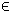## 2LP Problem

The general formulation in TOMLAB for a linear programming problem is:
 min x
f(x) = cT x

s/t
 xL ≤ x ≤ xU bL ≤ A x ≤ bU
(1)
where c, x, xL, xURn, ARm1 × n, and bL,bURm1. Equality constraints are defined by setting the lower bound to the upper bound.

Example problem:

 min x1,x2
f(x1,x2) = −7x1−5x2

s/t
 x1+2x2 ≤ 6 4x1+x2 ≤ 12 x1,x2 ≥ 0
(2)

The following file defines this problem in TOMLAB.

File: tomlab/quickguide/lpQG.m

Open the file for viewing, and execute lpQG in Matlab.
```% lpQG is a small example problem for defining and solving
% linear programming problems using the TOMLAB format.

Name  = 'lpQG';     % Problem name, not required.
c     = [-7 -5]';   % Coefficients in linear objective function
A     = [ 1  2
4  1 ];   % Matrix defining linear constraints
b_U   = [ 6 12 ]';  % Upper bounds on the linear inequalities
x_L   = [ 0  0 ]';  % Lower bounds on x

% x_min and x_max are only needed if doing plots
x_min = [ 0  0 ]';
x_max = [10 10 ]';

% b_L, x_U and x_0 have default values and need not be defined.
% It is possible to call lpAssign with empty [] arguments instead
b_L   = [-inf -inf]';
x_U   = [];
x_0   = [];

% Assign routine for defining an LP problem. This allows the user
% to try any solver, including general nonlinear solvers.
Prob = lpAssign(c, A, b_L, b_U, x_L, x_U, x_0, Name,...
[], [], [], x_min, x_max, [], []);

% Calling driver routine tomRun to run the solver.
% The 1 sets the print level after optimization.

% Result.x_k contains the optimal decision variables.
% Result.f_k is the optimal value.

Result = tomRun('pdco', Prob, 1);

%Result = tomRun('lpSimplex', Prob, 1);
%Result = tomRun('minos', Prob, 1);
%Result = tomRun('snopt', Prob, 1);
%Result = tomRun('conopt', Prob, 1);
%Result = tomRun('knitro', Prob, 1);
%Result = tomRun('cplex', Prob, 1);
%Result = tomRun('xpress-mp', Prob, 1);```